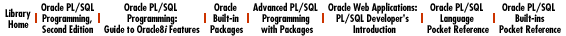start page | rating of books | rating of authors | reviews | copyrights

#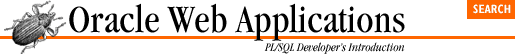## 8.2 Example 1: An Anonymous Survey

Our first application will let users fill out anonymous surveys using a web browser. Surveys and opinion polls are some of the most common web applications, and usually consist of a list of questions. Users respond by selecting an answer from a small list of options. Most systems also have an option that lets users see the tabulated results for each survey.

This section walks through a simple process you can use to create a generic survey system. Our first step is to design a storyboard to define each screen in the system. This sketch helps us in the next step: designing a generic data model that we can use to construct each page. Our last step is to actually code the system.

### 8.2.1 Storyboard

In a typical survey application, the first screen presents a list of all available surveys. There are usually two options for each survey: to answer it or to view its tabulated results. If the user decides to answer a survey, she's presented with a bunch of questions and a corresponding list of possible answers. She then answers the questions and presses "Submit" to save the responses in a database table. If the user chooses to view the results of a survey, she's presented with a table summarizing all the previous responses. Figure 8.2 is a simple storyboard that captures these functions.

#### Figure 8.2: The storyboard for the anonymous survey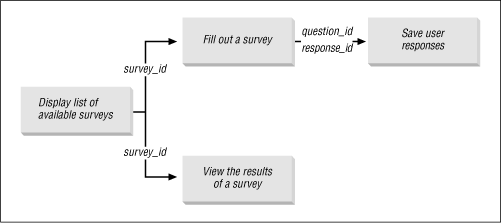### 8.2.2 Data Model

We can use the storyboard to design a data model. The first storyboard screen tells us that we need some sort of table to hold the survey list. The screen used to respond to a survey suggests three more tables. The first table holds the text of each question, the second holds the possible answers for each question, and the third holds the actual user responses. The "view results" screen queries these tables.

Figure 8.3 shows a data model that uses these four tables. The SURVEY table contains information about the survey itself, such as its name, description, and the date range during which it is available. The rows in QUESTIONS represent the individual questions on a survey. ANSWERS defines the list of valid responses for each question. The final table, RESPONSES, holds the actual responses given by the respondents.

#### Figure 8.3: A data design for the anonymous survey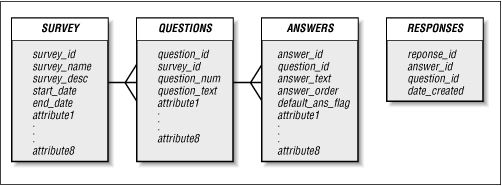### 8.2.3 Implementation Notes

Since the survey application is a fairly simple system, we can implement it using a single package, which we'll call EMP_SURVEY. We'll store all the application objects, including code and tables, in a schema named SURVEY.

### 8.2.4 The EMP_SURVEY Package

Now that we've got the basic screen layout and data model, we're finally ready to develop the actual package. Table 8.1 shows the five procedures contained in EMP_SURVEY.

Table 8.1: The EMP_SURVEY Package

Procedure

Parameters

Description

display_survey_list

None

Generates an HTML list of available surveys, with descriptions.

i_survey_id IN VARCHAR2

Creates the HTML form that allows a user to respond to the survey.

process_survey

question IN response_array

DEFAULT emp_survey.no_

response

response IN response_array

DEFAULT emp_survey.no_

response

Inserts the user's answers into the RESPONSES table.

view_results

i_survey_id IN VARCHAR2

Prints each question in the survey. Together, view_results and print_answers tabulate the results for the survey.

i_question_id IN VARCHAR2

Prints the corresponding summary information for a question. Together, view_results and print_answers tabulate the results for the survey.

Like all packages, EMP_SURVEY requires a specification and a body.

#### 8.2.4.1 Specification

We can translate the storyboard almost directly into a package specification. The four boxes on the diagram, each of which represents a screen in the system, indicate that we'll need at least four procedures. The lines connecting the boxes give us the formal parameter list for each procedure. Not everything is on the storyboard, though; we'll also need to declare an array to hold the user's survey answers. We'll discuss how to use this when we write the program to create the form.

The code for the EMP_SURVEY package is as follows:

`/* Formatted by PL/Formatter v.1.1.13 */ CREATE OR REPLACE PACKAGE emp_survey AS        /*    || Datatype used to hold the responses to the survey    */    TYPE response_array IS TABLE OF VARCHAR2(20)       INDEX BY BINARY_INTEGER;    no_response response_array;        -- Display the list of available surveys    PROCEDURE display_survey_list;        -- Display the form so that the user can respond    PROCEDURE answer_survey (i_survey_id IN VARCHAR2);        -- Save the responses    PROCEDURE process_survey (       question IN response_array DEFAULT emp_survey.no_response,       response IN response_array DEFAULT emp_survey.no_response       );        -- Display the results of a survey    PROCEDURE view_results (i_survey_id IN VARCHAR2);  END;`

#### 8.2.4.2 Body

With the basic design complete, all that's left is to fill in the pieces. In the survey system, this consists largely of building screens based on the information in our data model. Recalling the specification, we have to write five procedures for the package body: display_survey_list, answer_survey, process_survey, view_results, and print_answers.

#### 8.2.4.2.1 The display_survey_list procedure

The first procedure, display_survey_list, generates the first page of the storyboard. This page presents an HTML list of all available surveys along with their descriptions. Each survey requires two hyperlinks: one to link to the "response" page and one to link to the "view results" page.

The following procedure uses the SURVEY table to create the list. For each row in the table, the procedure creates a new HTML list item based on the survey_name and survey_desc columns. It also creates the two hyperlinks that include the survey_id as a parameter in the query string:

`PROCEDURE display_survey_list IS    -- Create cursor of all surveys that are active    -- Done by testing the start_date and end_date columns    -- of the SURVEY table    CURSOR survey_cur    IS       SELECT *         FROM survey        WHERE SYSDATE BETWEEN start_date AND end_date;    survey_rec survey_cur%ROWTYPE;    rec_count NUMBER DEFAULT 0;  BEGIN    HTP.print ('<title>Available Surveys</title>');    HTP.print ('<body bgcolor=white>');    HTP.print ('<ol>');   -- Begin an ordered list    OPEN survey_cur;    LOOP       FETCH survey_cur INTO survey_rec;       EXIT WHEN survey_cur%notfound;       HTP.print ('<li>');       HTP.bold (survey_rec.survey_name);       HTP.print ('<br>');       HTP.print ('<i>' || survey_rec.survey_desc || '</i><br>');       -- Put an anchor to take the survey       HTP.anchor (          'emp_survey.answer_survey?i_survey_id=' ||          survey_rec.survey_id,          'Take the survey'       );       -- Put an anchor to view the results       HTP.anchor (          'emp_survey.view_results?i_survey_id=' ||          survey_rec.survey_id,          'View the results'       );       HTP.print ('<p></li>');    END LOOP;    HTP.print ('</ol>');    CLOSE survey_cur; END display_survey_list;	`

Figure 8.4 shows the procedure's output.

#### Figure 8.4: The main screen of the survey applicationThe next procedure creates the HTML form that allows a user to respond to the survey. answer_survey has one parameter, survey_id, to indicate the survey the user selected on the preceding screen. answer_survey uses the rows of the QUESTION table for the specified survey to build corresponding rows in a two-column table. The first column in the table holds the question's text. The second column holds two form ``` <input>``` elements. The first element, a hidden field containing the question's ID, is needed to associate the user's answer with a corresponding question. The second element, created by the OWA_UTIL.LISTPRINT procedure, is a selectable list of the question's possible answers as they appear in the ANSWERS table. When the user submits the form, both the hidden field and the response field are passed as parameter arrays to the process_survey procedure.

The code for this procedure is as follows:

`PROCEDURE answer_survey (i_survey_id IN VARCHAR2) IS    CURSOR q_cur    IS       SELECT *         FROM questions        WHERE survey_id = i_survey_id        ORDER BY question_num;        q_rec q_cur%ROWTYPE;    stmt VARCHAR2(500);  BEGIN    HTP.title ('Survey');    HTP.print ('<body bgcolor=white>');    HTP.print ('<form action=emp_survey.process_survey>');    HTP.print ('<table width=80%>');    OPEN q_cur;    LOOP       FETCH q_cur INTO q_rec;       EXIT WHEN q_cur%notfound;       -- Start a new row in the HTML table       HTP.print ('<tr>');       -- Print the question in column 1       HTP.print ('<th align=left valign=top>');       HTP.print (q_rec.question_text);       HTP.print ('</th>');       -- Put the question_id and select list in column 2       HTP.print ('<td>');       -- Put the question_id in as a hidden field       HTP.formhidden (          cname => 'question',          cvalue => q_rec.question_id       );       -- Use owa_util.listprint to build the LOV for the answer        stmt :=          'select answer_id, answer_text, default_ans_flag ';       stmt := stmt || ' from survey.answers where';       stmt := stmt || ' question_id = ' || q_rec.question_id;       stmt := stmt || ' order by answer_order';       OWA_UTIL.listprint (stmt, 'response', 4, FALSE);       HTP.print ('</td>');       HTP.print ('</tr>');    END LOOP;    HTP.print ('</table>');    HTP.formsubmit;    CLOSE q_cur; END;`

Figure 8.5 shows the output of this procedure for a sample survey.

#### Figure 8.5: The form used to answer a survey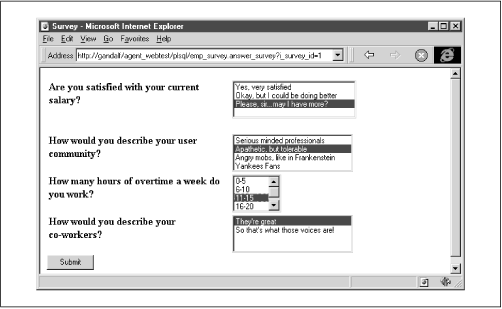#### 8.2.4.2.3 The process_survey procedure

The third procedure, process_survey, inserts the user's answers into the RESPONSES table. It accepts two response_array parameters (see the specification), question and response. For each question on the answer form, these parameters hold (respectively) the ID of the question as defined in QUESTIONS and the ID of the user's corresponding answer as defined in ANSWERS.

The procedure loops through each element, inserting a new row in the RESPONSES table for each question. The procedure calls the display_survey_list procedure to return the user to the main screen:

`PROCEDURE process_survey (    question IN response_array DEFAULT emp_survey.no_response,    response IN response_array DEFAULT emp_survey.no_response    ) IS        count NUMBER DEFAULT 0;  BEGIN    HTP.title ('Saving Responses');    HTP.print ('<body bgcolor=white>');    -- COUNT attribute available in PL/SQL 2.3    FOR count IN 1 .. question.count    LOOP       INSERT INTO responses (response_id,question_id,answer_id)          VALUES (             response_seq.nextval,             question (item_count),             response (item_count)           );    END LOOP;    HTP.print ('<h1>Values Saved</h1><hr>');    display_survey_list; END;`

#### 8.2.4.2.4 The view_results procedure

The last procedure in the application tabulates the results for the survey specified by the survey_id parameter. The procedure is split into two parts: a main procedure (view_results) to print each question and a secondary procedure (print_answers) to print a corresponding summary. The code for the main procedure is as follows:

`PROCEDURE view_results (i_survey_id IN VARCHAR2) IS    CURSOR q_cur    IS       SELECT *         FROM questions        WHERE survey_id = i_survey_id        ORDER BY question_num;    q_rec q_cur%ROWTYPE; BEGIN    HTP.title ('Survey Responses');    HTP.print ('<body bgcolor=white>');    HTP.print ('<ol>');    OPEN q_cur;    LOOP       FETCH q_cur INTO q_rec;       EXIT WHEN q_cur%notfound;       HTP.print ('<li>');       HTP.print ('<b>' || q_rec.question_text || '</b>');       HTP.print ('<br>');       -- Call another procedure to generate the response summary       print_answers (q_rec.question_id);       HTP.print ('</td>');    END LOOP;    HTP.print ('</ol>');    CLOSE q_cur; END;`

The secondary procedure, print_answers, generates the summary information for a question. The procedure opens a cursor based on the ANSWERS table to retrieve all the answers for a particular question. It then queries the RESPONSES table to calculate the percentages for that response. The code for the procedure is as follows:

`PROCEDURE print_answers (i_question_id IN VARCHAR2) IS    CURSOR ans_cur    IS       SELECT *         FROM answers        WHERE question_id = i_question_id        ORDER BY answer_order;    ans_rec ans_cur%ROWTYPE;    total_responses NUMBER;    num_responses NUMBER; BEGIN    -- Fetch the total number of responses    SELECT COUNT (*)      INTO total_responses      FROM responses     WHERE question_id = i_question_id;    HTP.print ('<table width=50%>');    OPEN ans_cur;    LOOP       FETCH ans_cur INTO ans_rec;       EXIT WHEN ans_cur%notfound;       HTP.print ('<tr>');       -- Print question text       HTP.print ('<td nowrap>' ||                  ans_rec.answer_text ||                  '</td>');       -- Fetch and print number of responses       SELECT COUNT (*)         INTO num_responses         FROM responses        WHERE answer_id = ans_rec.answer_id;       HTP.print ('<td align=right>' ||                  ROUND (                     num_responses / total_responses * 100,                     2                  ) ||                  '%</td>');       HTP.print ('</tr>');    END LOOP;    HTP.print ('</table>');    CLOSE ans_cur; END;`

Figure 8.6 shows the combined results of these procedures.

#### Figure 8.6: A sample summary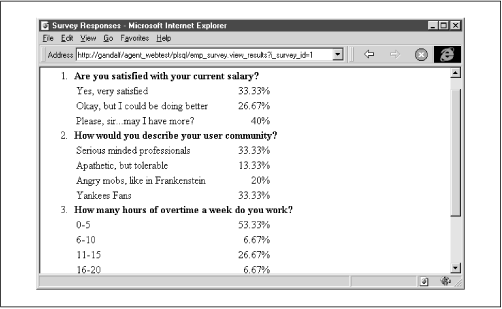### 8.2.5 Security Privileges

Our last formal step is to make the package accessible to the user's web browser. Rather than creating a new PL/SQL agent for the SURVEY schema, we can make the package available to an existing agent's schema (in this case, WEBTEST). This makes the system more secure and reduces maintenance for the webmaster. Here are the steps to follow:

2. Grant EXECUTE privileges on the EMP_SURVEY package to the agent account (WEBTEST).

3. Connect to the agent account (again, WEBTEST).

4. Create a synonym called EMP_SURVEY for survey.emp_survey.

Figure 8.7 shows how these commands are used in SQL*Plus.

#### Figure 8.7: Using SQL*Plus to grant privileges to the PL/SQL agent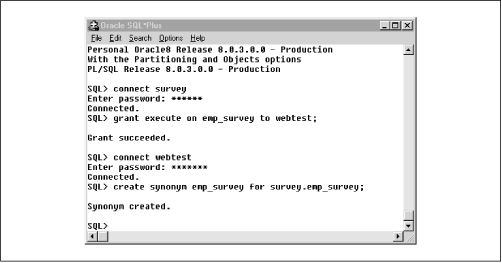### 8.2.6 Summary

Developing the anonymous survey has taught us several things about web development. First, we have seen that a storyboard is a good place to start when faced with a new application. We can use the information on our diagram to define how the user will navigate, get a good idea of the database tables we'll need, and get a jump-start on defining the package specification. Second, this example has illustrated how to use parameter arrays to pass multiple field values, as well as how (and under what circumstances) you should use hidden fields. We'll expand these ideas further in the next sample application.8.1 Designing a Web Application8.3 Example 2: A Discussion Forum